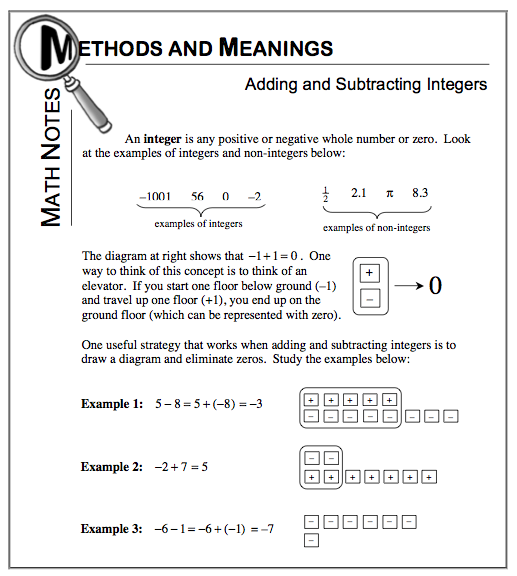### Home > AC > Chapter 1 > Lesson 1.1.3 > Problem1-22

1-22.

Compute Without a calculator. Homework Help ✎

1. $−15+7$

One strategy is to draw a diagram and eliminate zeros. Refer to the Math Notes box at right from lesson 1.1.3 to help set up the diagram.$-15+7=-8$

1. $8−(−21)$

$8-(-21)=8+21$

1. $−12−(−4)$

1. $−9+(−13)$

1. $−50−30$

Use the strategies shown in problems (a) and (b) to help you solve.

1. $3−(−9)$

1. $−75−(−75)$

1. $(−3)+6$

1. $9+(−14)$

1. $28−(−2)$

1. $−3+(−2)+5$

Separate the problem into two parts. Solve the first part and then attempt the remaining part of the problem.

1. $3+2+5$